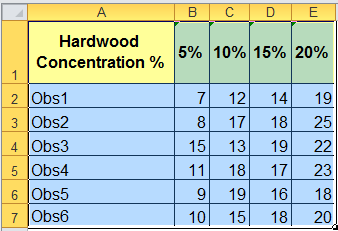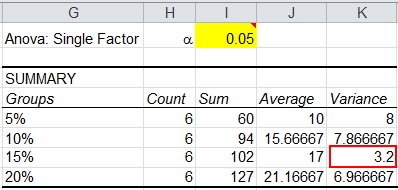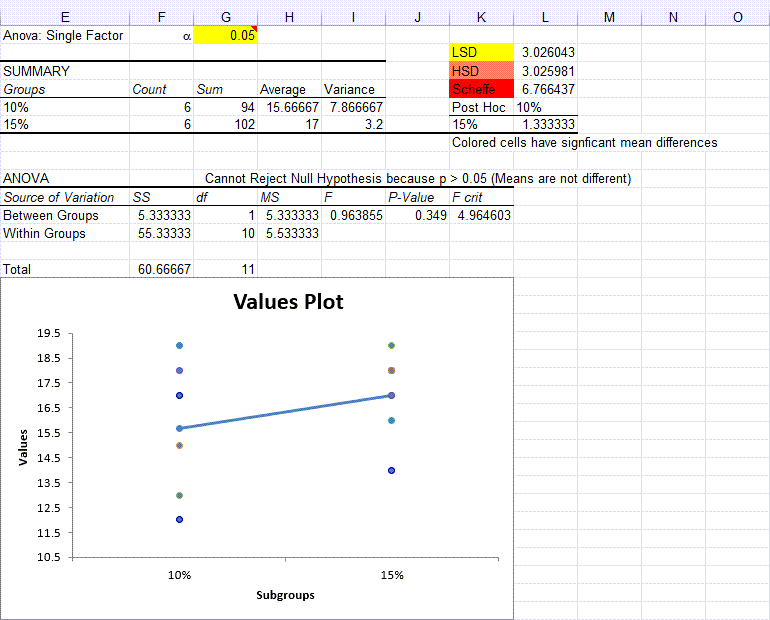# One-Way ANOVA (ANalysis Of VAriance)

## QI Macros can do it for you!

### Create One-Way ANOVA using QI Macros:

2. Click on QI Macros menu > Statistical Tools > ANOVA > ANOVA One-Way
3. QI Macros will do the math and analysis for you.

## One-Way ANOVA Example

Imagine you manufacture paper bags and you want to improve the tensile strength of the bag. You suspect that changing the concentration of hardwood in the bag will change the tensile strength. You measure the tensile strength in pounds per square inch (PSI). So, you decide to test this at 5%, 10%, 15% and 20% hardwood concentration levels. These "levels" are also called "treatments."

Since we are only evaluating a single factor (hardwood concentration) this is called one-way ANOVA.

To conduct the one-way ANOVA test, you need to randomize the trials (assumption #1). Imagine that we've conducted these trials at each of the four levels of hardwood concentration.

#### To perform one way Analysis of Variance in Excel using QI Macros follow these steps:

1. Just click and drag over the data to select it.2. Now click on QI Macros menu, Statistical Tools and ANOVA: Single factor.
3.4. QI Macros will prompt you for the significance level you desire.  The default is 0.05 (95% confident).
5. QI Macros will perform the calculations and analyze the results for you.### Interpreting ANOVA One Way Test Results Yourself

QI Macros automatically compares the p value to a, but you might want to know how to do this manually. The "null" hypothesis assumes that there is no difference between the hardwood concentrations.

H0: Mean1 = Mean2 = Mean3 = Mean4

The alternate hypothesis is that at least one of the means is different:

Ha: At least one of the means is different
 If Then test statistic > critical value  (i.e. F> Fcrit) Reject the null hypothesis (Means are Different/Means are not the Same) test statistic < critical value  (i.e. F< Fcrit) Cannot Reject the null hypothesis (Accept null hypothesis) (Means are not Different/Means are the Same) p value < a Reject the null hypothesis p value > a Cannot Reject the null hypothesis Accept null hypothesis)

The P-value of 0.000 is less than the significance level (0.01), so we can reject the null hypothesis and safely assume that hardwood concentration affects tensile strength.

F (19.60521) is greater than F crit (4.938193), so again, we can reject the null hypothesis.

Now we can look at the average tensile strength and variances:The average tensile strength increases, but we cannot say for certain which means differ. The variance at the 15% level looks substantially lower than the other levels. We might need to do additional analysis.

If we reran the one way ANOVA test with just 10% and 15%, we'd discover there is no statistically significant difference between the two means.The P value (0.349) is greater than the significance level (0.05), so we "Cannot Reject the Null Hypothesis (Accept the Null Hypothesis)" that the means are equivalent. And F (0.963855) is less than F crit (4.964603) so we "Cannot Reject the Null Hypothesis (Accept the Null Hypothesis)."

Based on this analysis, if we were aiming for a tensile strength of 15 PSI or greater, the 10% level might be more cost effective.

This example came from Montgomery's Intro to SPC book.

### Why Choose QI Macros Statistical Software for Excel?### Easy to Use

• Works Right in Excel
• Interprets p-values for You
• Accurate No-Worry Results
• Free Training Anytime### Proven and Trusted

• 100,000 Users in 80 Countries
• Celebrating 20th Anniversary
• Five Star CNET Rating - Virus Free### Affordable

• Only \$349 USD
Quantity Discounts Available
• No annual fees
• Free Technical Support